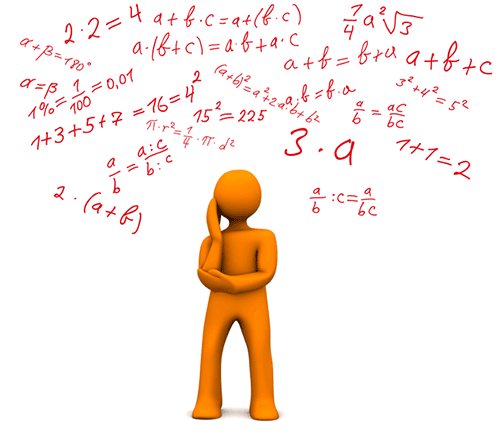## This module is for Algebra 1, Grades 7-12 Welcome

Numbers form a basis for the language of mathematics and science. Fractions, decimals, square roots, absolute values, negative numbers… all kinds of numbers are used! To make better sense of numbers, mathematicians categorize numbers into number systems based upon numbers’ defining characteristics and properties. In this module, you will explore the various number systems. By the end of the module, you will be able to explain how numbers in different number systems relate to one another.

### Module Objective

By the end of this module, you will be able to:

• Explain why the sum or product of two rational numbers is rational; that the sum of a rational number and an irrational number is irrational; and that the product of a nonzero rational number and an irrational number is irrational.### Skills

• Find the sum and the product of two rational numbers.
• Identify the sum or product of two rational numbers as a rational number.
• Find the sum of a rational number and an irrational number.
• Identify the sum of a rational number and an irrational number as an irrational number.
• Find the product of a nonzero rational number and an irrational number.
• Identify the product of a nonzero rational number and an irrational number as an irrational number.